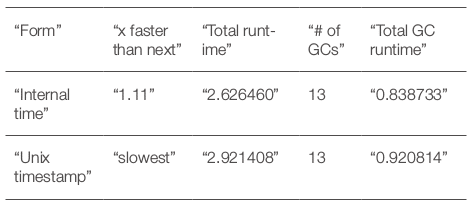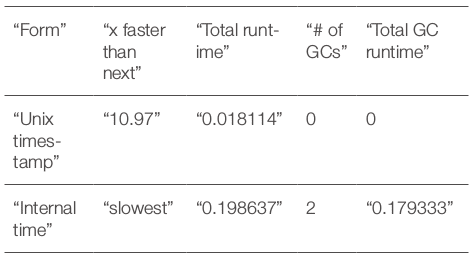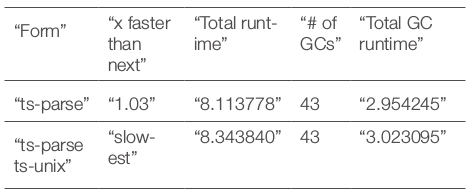# The Emacs Series Exploring ts.el

0
1040In this article, the author reviews the ts.el date and time library for Emacs. Written by Adam Porter, ts.el is still in the development phase and has been released under the GNU General Public License v3.0.

The ts.el package uses intuitive names for date and time functions. It internally uses UNIX timestamps and depends on both the ‘dash’ and ‘s’ Emacs libraries. The parts of the date are computed lazily and also cached for performance. The source code is available at https://github.com/alphapapa/ts.el. In this article, we will explore the API functions available from the ts.el library.

Installation
The package does not have a tagged release yet; hence, you should download it from https://github.com/alphapapa/ts.el/blob/master/ts.el and add it to your Emacs load path to use it. You should also have the ‘dash’ and ‘s’ libraries installed and loaded in your Emacs environment. You can then load the library using the following command:

`(require ‘ts)`

Usage
Let us explore the various functions available to retrieve parts of the date from the ts.el library. When the examples were executed, the date was ‘Friday July 5, 2019’. The ts-dow function can be used to obtain the day of the week, as shown below:

```(ts-dow (ts-now))
5```

ts-now is a Lisp construct that returns a timestamp set. It is defined in ts.el as follows:

```(defsubst ts-now ()
“Return `ts’ struct set to now.”
(make-ts :unix (float-time)))```

The day of the week starts from Monday (1) and hence Friday has the value of 5. An abbreviated form of the day can be fetched using the ts-day-abbr function. In the following example, ‘Friday’ is shortened to‘Fri’.

```(ts-day-abbr (ts-now))
"Fri"```

The day of the week in full form can be obtained using the ts-day-name function, as shown below:

```(ts-day-name (ts-now))
“Friday”```

The twelve months from January to December are numbered from 1 to 12 respectively. Hence, for the month of July, the index number is 7. This numeric value for the month can be retrieved using the ‘ts-month’ API. For example:

```(ts-month (ts-now))
7```

If you want a three-character abbreviation for the month’s name, you can use the ts-month-abbr function as shown below:

```(ts-month-abbr (ts-now))
“Jul”```

The ts-month-name function can be used to obtain the full name of the month. For example:

```(ts-month-name (ts-now))
“July”```

The day of the week starts from Monday and has an index 1, while Sunday has an index 7. If you need the numeric value for the day of the week, use the ts-day function as indicated below:

```(ts-day (ts-now))
5```

The ts-year API returns the year. In our example, it is ‘2019’ as shown below:

```(ts-year (ts-now))
2019```

The hour, minute and seconds can be retrieved using the ts-hour, ts-minute and ts-second functions, respectively. Examples of these functions are given below:

```(ts-hour (ts-now))
18

(ts-minute (ts-now))
19

(ts-second (ts-now))
5```

The UNIX timestamps are in UTC, by default. The ts-tz-offset function returns the offset from UTC. The Indian Standard Time (IST) is five-and-a-half-hours ahead of UTC and hence this function returns ‘+0530’ as shown below:

```(ts-tz-offset (ts-now))
"+0530"```

The ts-tz-abbr API returns an abbreviated form of the time zone. In our case, ‘IST’ is returned for the Indian Standard Time.

```(ts-tz-abbr (ts-now))
"IST"```

The ts-adjustf function applies the time adjustments passed to the timestamp and the ts-format function formats the timestamp as a string. A couple of examples are given below:

```(let ((ts (ts-now)))
(ts-format nil ts))
“2019-07-06 18:23:24 +0530”

(let ((ts (ts-now)))
(ts-adjustf ts ‘year 1 ‘month 3 ‘day 5)
(ts-format nil ts))
“2020-10-10 18:24:07 +0530”```

You can use the ts-dec function to decrement the timestamp. For example:

```(ts-day-name (ts-dec ‘day 1 (ts-now)))
“Thursday”```

The threading macro syntax can also be used with the ts-dec function as shown below:

```(->> (ts-now) (ts-dec ‘day 2) ts-day-name)
“Wednesday”```

The UNIX epoch is the number of seconds that have elapsed since January 1, 1970 (midnight UTC/GMT). The ts-unix function returns an epoch UNIX timestamp as illustrated below:

```(ts-unix (ts-adjust ‘day -2 (ts-now)))
1562158551.0 ;; Wednesday, July 3, 2019 6:25:51 PM GMT+05:30```

An hour has 3600 seconds and a day has 86400 seconds. You can compare epoch timestamps as shown in the following example:

```(/ (- (ts-unix (ts-now))
86400)
4```

The ts-difference function returns the difference between two timestamps, while the ts-human-duration function returns the property list (plist) values of years, days, hours, minutes and seconds. For example:

```(ts-human-duration
(ts-difference (ts-now)
(ts-dec ‘day 3 (ts-now))))
(:years 0 :days 3 :hours 0 :minutes 0 :seconds 0)```

A number of aliases are available for the hour, minute, second, year, month and day format string constructors. A few examples are given below:

```(ts-hour (ts-now))
18
(ts-H (ts-now))
18

(ts-minute (ts-now))
46
(ts-min (ts-now))
46
(ts-M (ts-now))
46

(ts-second (ts-now))
16
(ts-sec (ts-now))
16
(ts-S (ts-now))
16

(ts-year (ts-now))
2019
(ts-Y (ts-now))
2019

(ts-month (ts-now))
7
(ts-m (ts-now))
7

(ts-day (ts-now))
5
(ts-d (ts-now))
5```

You can parse a string into a timestamp object using the ts-parse function. For example:

```(ts-format nil (ts-parse “Fri Dec 6 2019 18:48:00”))
“2019-12-06 18:48:00 +0530”```

You can also format the difference between two timestamps in a human readable format as shown in the following example:

```(ts-human-format-duration
(ts-difference (ts-now)
(ts-adjust ‘day -1 ‘hour -3 ‘minute -2 ‘second -4 (ts-now))))
“1 days, 3 hours, 2 minutes, 4 seconds”```

The timestamp comparator operations are also defined in ts.el. The ts< function compares if one epoch UNIX timestamp is less than the other. Its definition is as follows:

```(defun ts< (a b)
“Return non-nil if timestamp A is less than timestamp B.”
(< (ts-unix a) (ts-unix b)))```

In the example given below, the current timestamp is not less than the previous day and hence it returns nil.

```(ts< (ts-now) (ts-adjust ‘day -1 (ts-now)))
nil```

Similarly, we have other comparator functions like ts>, ts=, ts>= and ts<=. A few examples of these function use cases are given below:

```(ts> (ts-now) (ts-adjust ‘day -1 (ts-now)))
t

(ts= (ts-now) (ts-now))
nil

(ts>= (ts-now) (ts-adjust ‘day -1 (ts-now)))
t

(ts<= (ts-now) (ts-adjust ‘day -2 (ts-now)))
nil```

Benchmarking
A few performance tests can be conducted to compare the Emacs internal time values versus the UNIX timestamps. The benchmarking tests can be executed by including the bench-multi macro and bench-multi-process-results function available from https://github.com/alphapapa/emacs-package-dev-handbook in your Emacs environment.
You will also need to load the dash-functional library to use the -on function.

`(require ‘dash-functional)`

The following tests have been executed on an Intel(R) Core(TM) i7-3740QM CPU at 2.70GHz with eight cores, 16GB RAM and running Ubuntu 18.04 LTS.

Formatting
The first benchmarking exercise is to compare the formatting of the UNIX timestamp and the Emacs internal time. The Emacs Lisp code to run the test is shown below:

```(let ((format “%Y-%m-%d %H:%M:%S”))
(bench-multi :times 100000
:forms ((“Unix timestamp” (format-time-string format 1544311232))
(“Internal time” (format-time-string format ‘(23564 20962 864324 108000))))))```

The output appears as an s-expression:

```((“Form” “x faster than next” “Total runtime” “# of GCs” “Total GC runtime”)
hline

(“Internal time” “1.11” “2.626460” 13 “0.838733”)
(“Unix timestamp” “slowest” “2.921408” 13 “0.920814”))```

The abbreviation ‘GC’ refers to garbage collection. A tabular representation of the above results is given below:We observe that formatting the internal time is slightly faster.

Getting the current time
The functions to obtain the current time can be compared using the following test:

```(bench-multi :times 100000
:forms ((“Unix timestamp” (float-time))
(“Internal time” (current-time))))```

The results are shown below:We observe that using the Unix timestamp is faster.

Parsing
The third benchmarking exercise is to compare parsing functions on a date timestamp string. The corresponding test code is given below:

```(let* ((s “Wed 10 Jul 2019”))
(bench-multi :times 100000
:forms ((“ts-parse” (ts-parse s))
(“ts-parse ts-unix” (ts-unix (ts-parse s))))))```

The ts-parse function is slightly faster than the ts-parse ts-unix function, as seen in the results:A new timestamp versus blanking fields
The last performance comparison is between creating a new timestamp and blanking the fields. The relevant test code is as follows:

```(let* ((a (ts-now)))
(bench-multi :times 100000
:ensure-equal t
:forms ((“New” (let ((ts (copy-ts a)))
(setq ts (ts-fill ts))
(make-ts :unix (ts-unix ts))))
(“Blanking” (let ((ts (copy-ts a)))
(setq ts (ts-fill ts))
(ts-reset ts))))))```

The output of the benchmarking exercise is given below:We observe that creating a new timestamp is slightly faster than blanking the fields.